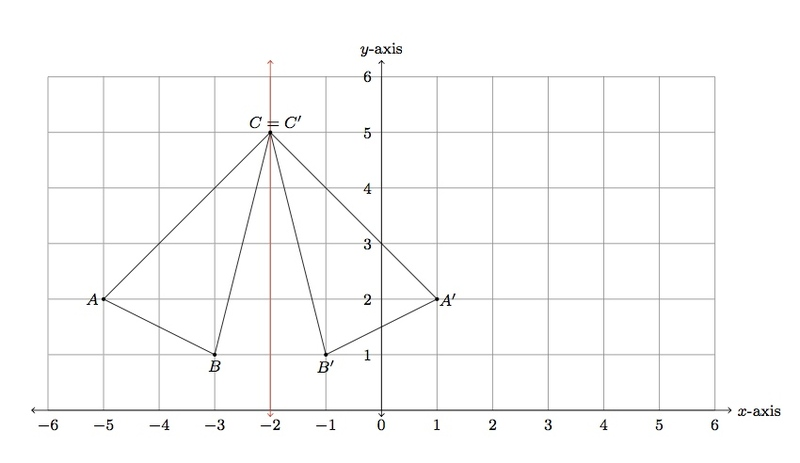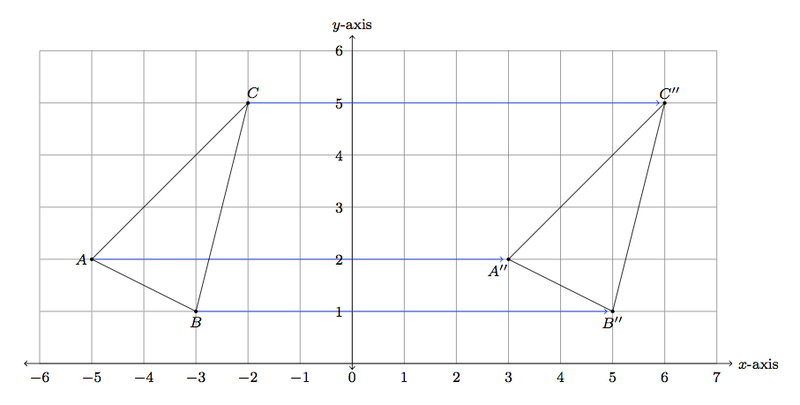# Reflecting reflections

Alignments to Content Standards: 8.G.A.3

Below is a picture of a triangle on a coordinate grid:1. Draw the reflection of $\triangle ABC$ over the line $x = -2$. Label the image of $A$ as $A^\prime$, the image of $B$ as $B^\prime$ and the image of $C$ as $C^\prime$.
2. Draw the reflection of $\triangle A^{\prime}B^{\prime}C^\prime$ over the line $x = 2$. Label the image of $A^\prime$ as $A^{\prime \prime}$, the image of $B^\prime$ as $B^{\prime \prime}$ and the image of $C^\prime$ as $C^{\prime \prime}$.
3. What single rigid transformation of the plane will map $\triangle ABC$ to $\triangle A^{\prime \prime}B^{\prime \prime}C^{\prime \prime}$? Explain.

## IM Commentary

The goal of this task is to give students an opportunity to experiment with reflections of triangles on a coordinate grid. Students are not prompted in the question to list the coordinates of the different triangle vertices but this is a natural extension of the task. Another valid approach would use the geometry of the coordinate grid without making explicit reference to the coordinates of the triangle vertices. This approach is also valuable in preparation for the coordinate-free transformation approach to reflections which will be seen in high school.

This task examines the composition of successive reflections about parallel lines. The result is a translation. If we denote by $L_1$ the first line, $x = -2$, and by $L_2$ the second line, $x = 2$, then the composition of reflection about $L_1$ followed by reflection about $L_2$ is a translation by twice the distance between $L_1$ and $L_2$ (in the direction going from $L_1$ to $L_2$).

If the teacher wishes to give students further work with reflections, a good question would be to have them first reflect $\triangle ABC$ about $x = 2$ and then about $x = -2$. This is also a translation but is it the same translation as in part (c) of the problem? This is an important example of two transformations (reflections of the plane) which do not commute: that is, the order in which the reflections are implemented changes the outcome.

The teacher may also wish to examine the case of successive reflections about lines which are not parallel: for example the line $y = x$ and the $x$-axis. Here again the order in which the reflections are performed will matter and the result will be a rotation. A little more work in this direction shows the remarkable fact that all rigid motions of the plane can be written in terms of reflections.

## Solution

1. Below is a picture of triangle $ABC$ and its reflection about $x = -2$, triangle $A^{\prime}B^{\prime}C^\prime$.Note that $C^\prime = C$ becuase $C$ lies on the line $x = -2$. Points on $x = -2$ are not influenced by the reflection. For points which are not on $x = -2$, the $y$-coordinate remains the same. To see why, consider the point $A = (-5,2)$. This is on the line $y = 2$ which is perpendicular to the line $x = -2$. If we take $A^\prime = (1,2)$ then $x = -2$ is the perpendicular bisector of $\overline{AA^\prime}$ and this means that $A^\prime$ is the reflection of $A$ over the line $x = -2$. Similarly, $B^\prime = (-1,1)$ because $x = -2$ is the perpendicular bisector of $\overline{BB^\prime}$.

2. Below is the picture of triangle $ABC$ and its reflection $A^{\prime}B^\prime C^\prime$ together with the reflection of $A^{\prime}B^{\prime}C^\prime$ over the line $x = 2$.As in part (a), the $y$-coordinates of $A^{\prime \prime}$, $B^{\prime \prime}$, and $C^{\prime \prime}$ are the $y$-coordinates of $A,B,$ and $C$. Also as in part (a), the $x$-coordinate of $A^{\prime \prime}$, $B^{\prime \prime}$, and $C^{\prime \prime}$ is selected so that the line $x = 2$ is the perpendicular bisector of segments $\overline{A^{\prime}A^{\prime \prime}}$, $\overline{B^{\prime}B^{\prime \prime}}$, and $\overline{C^{\prime}C^{\prime \prime}}$.

3. The picture below shows triangles $ABC$ and $A^{\prime \prime}B^{\prime \prime}C^{\prime \prime}$ and the blue arrows indicate that to transform $\triangle ABC$ to $\triangle A^{\prime \prime}B^{\prime \prime}C^{\prime \prime}$ we can translate by $8$ units to the right. So the composition of these two reflections about $x = -2$ and $x = 2$ is a translation by $8$ units to the right.Further experimentation with reflections about parallel lines will show that the fact that the translation here moves to the right comes from the fact that the line $x = 2$ is to the right of $x = -2$. The fact that it is a translation by $8$ units is related to the fact that $x = 2$ is one half of this, or $4$ units, to the right of $x = -2$.